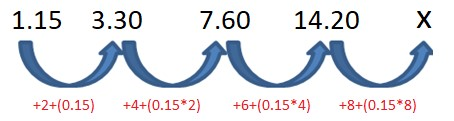# QOTD-Logical Reasoning-Analogy and Number Series

2019-06-29 | Team PendulumEdu

The numbers given below follows a certain pattern. Study the pattern and choose the number which will replace the question mark?

1.15, 3.30, 7.60, 14.20, ?

A. 22.40

B. 23.40

C. 21.60

D. 22.50

Consider the given series

1.15, 3.30, 7.60, 14.20, ?Thus,

X = 14.20 + 8 + (0.15 x 8)

X = 23.40

In the type of question where the numbers are in decimal try solving the question by adding or subtracting the decimal value or the multiples of the decimal values. As seen here the multiples of 0.15 are added with the elements of an increasing arithmetic progression of 2, 4, 6, 8 and so on.

Hence, (b) is the correct answer.

Register at PendulumEdu

View Our previous Question of the Day-GK-28-June-2019

Try Free Mock Test of IBPS PO

Try Free Mock Test of SSC CGL

Try Free Mock Test of SSC CHSL

Try Free Mock Test of SSC MTS

Try Free Mock Test of RRB NTPC0